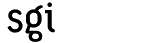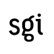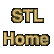# How to use the STL documentation

This site documents all of the components (classes, functions, and concepts) in the SGI Standard Template Library. Each page describes a single component, and also includes links to related components.

This documentation assumes a general familiarity with C++, especially with C++ templates. Additionally, you should read Introduction to the Standard Template Library before proceeding to the pages that describe individual components: the introductory page defines several terms that are used throughout the documentation.

## Classification of STL components

The STL components are divided into six broad categories on the basis of functionality: Containers, Iterators, Algorithms, Function Objects, Utilities, and Allocators; these categories are defined in the Introduction, and the Table of Contents is organized according to them.

The STL documentation classifies components in two ways.

1. Categories are a classification by functionality. The categories are:
• Container
• Iterator
• Algorithm
• Function Object
• Utility
• Allocator.
2. Component types are a structural classification: one based on what kind of C++ entity (if any) a component is. The component types are:
• Type (i.e. a struct or class)
• Function
• Concept (as defined in the Introduction).

These two classification schemes are independent, and each of them applies to every STL component; vector, for example, is a type whose category is Containers, and Forward Iterator is a concept whose category is Iterators.

Both of these classification schemes appear at the top of every page that documents an STL component. The upper left corner identifies the the component's category as Containers, Iterators, Algorithms, Function Objects, Utilities, Adaptors, or Allocators, and the upper right corner identifies the component as a type, a function, or a concept.

## Using the STL documentation

The STL is a generic library: almost every class and function is a template. Accordingly, one of the most important purposes of the STL documentation is to provide a clear description of which types may be used to instantiate those templates. As described in the Introduction, a concept is a generic set of requirements that a type must satisfy: a type is said to be a model of a concept if it satisfies all of that concept's requirements.

Concepts are used very heavily in the STL documentation, both because they directly express type requirements, and because they are a tool for organizing types conceptually. (For example, the fact that ostream_iterator and insert_iterator are both models of Output Iterator is an important statement about what those two classes have in common.) Concepts are used for the documentation of both types and functions.

### The format of a concept page

A page that documents a concept has the following sections.

• Summary: A description of the concept's purpose.
• Refinement of: A list of other concepts that this concept refines, with links to those concepts.
• Associated types: A concept is a set of requirements on some type. Frequently, however, some of those requirements involve some other type. For example, one of the Unary Function requirements is that a Unary Function must have an argument type; if F is a type that models Unary Function and f is an object of type F, then, in the expression f(x), x must be of F's argument type. If a concept does have any such associated types, then they are defined in this section.
• Notation: The next three sections, definitions, valid expressions, and expression semantics, present expressions involving types that model the concept being defined. This section defines the meaning of the variables and identifiers used in those expressions.
• Definitions: Some concepts, such as LessThan Comparable, use specialized terminology. If a concept requires any such terminology, it is defined in this section.
• Valid Expressions: A type that models a concept is required to support certain operations. In most cases, it doesn't make sense to describe this in terms of specific functions or member functions: it doesn't make any difference, for example, whether a type that models Input Iterator uses a global function or a member function to provide operator++. This section lists the expressions that a type modeling this concept must support. It includes any special requirements (if any) on the types of the expression's operands, and the expression's return type (if any).
• Expression Semantics: The previous section, valid expressions, lists which expressions involving a type must be supported; it doesn't, however, define the meaning of those expressions. This section does: it lists the semantics, preconditions, and postconditions for the expressions defined in the previous section.
• Complexity Guarantees: In some cases, the run-time complexity of certain operations is an important part of a concept's requirements. For example, one of the most significant distinctions between a Bidirectional Iterator and a Random Access Iterator is that, for random access iterators, expressions like p + n take constant time. Any such requirements on run-time complexity are listed in this section.
• Invariants: Many concepts require that some property is always true for objects of a type that models the concept being defined. For example, LessThan Comparable imposes the requirement of transitivity: if x < y and y < z, then x < z. Some such properties are "axioms" (that is, they are independent of any other requirements) and some are "theorems" (that is, they follow either from requirements in the expression semantics section or from other requirements in the invariants section).
• Models: A list of examples of types that are models of this concept. Note that this list is not intended to be complete: in most cases a complete list would be impossible, because there are an infinite number of types that could model the concept.
• Notes: Footnotes (if any) that are referred to by other parts of the page.

### The format of a type page

A page that documents a type has the following sections.

• Description. A summary of the type's properties.
• Example of use: A code fragment involving the type.
• Definition: A link to the source code where the type is defined.
• Template parameters: Almost all STL structs and classes are templates. This section lists the name of each template parameter, its purpose, and its default value (if any).
• Model of: A list of the concepts that this type is a model of, and links to those concepts. Note that a type may be a model of more than one concept: vector, for example, is a model of both Random Access Container and Back Insertion Sequence. If a type is a model of two different concepts, that simply means that it satisfies the requirements of both.
• Type requirements: The template parameters of a class template usually must satisfy a set of requirements. Many of these can simply be expressed by listing which concept a template parameter must conform to, but some type requirements are slightly more complicated, and involve a relationship between two different template parameters.
• Public base classes: If this class inherits from any other classes, they are listed in this section.
• Members: A list of this type's nested types, member functions, member variables, and associated non-member functions. In most cases these members are simply listed, rather than defined: since the type is a model of some concept, detailed definitions aren't usually necessary. For example, vector is a model of Container, so the description of the member function begin() in the Container page applies to vector, and there is no need to repeat it in the vector page. Instead, the Members section provides a very brief description of each member and a link to whatever page defines that member more fully.
• New Members: A type might have some members that are not part of the requirements of any of the concepts that it models. For example, vector has a member function called capacity(), which is not part of the Random Access Container or Back Insertion Sequence requirements. These members are defined in the New members section.
• Notes: Footnotes (if any) that are referred to by other parts of the page.

### The format of a function page

A page that documents a function has the following sections.

• Prototype: the function's declaration.
• Description: A summary of what the function does.
• Definition: A link to the source code where the function is defined.
• Requirements on types: Most functions in the STL are function templates. This section lists the requirements that must be satisfied by the function's template parameters. Sometimes the requirements can simply be expressed by listing which concept a template parameter must conform to, but sometimes they are more complicated and involve a relationship between two different template parameters. In the case of find, for example, the requirements are that the parameter InputIterator is a model of Input Iterator, that the parameter EqualityComparable is a model of Equality Comparable, and that comparison for equality is possible between objects of type EqualityComparable and objects of InputIterator's value types.
• Preconditions: Functions usually aren't guaranteed to yield a well-defined result for any possible input, but only for valid input; it is an error to call a function with invalid input. This section describes the conditions for validity.
• Complexity: Guarantees on the function's run-time complexity. For example, find's run-time complexity is linear in the length of the input range.
• Example of use: A code fragment that illustrates how to use the function.
• Notes: Footnotes (if any) that are referred to by other parts of the page.